# ILP Part 14 – Comparing real numbers

This is the fourteenth part of the ILP series. For your convenience you can find other parts in the table of contents in Part 1 – Boolean algebra

We already know how to compare integer values and today we are going to extend this method to be able to compare real numbers.

# Introduction

Comparing real numbers is a bit tricky. We need to remember that computers usually implement non-integer values using IEEE floating point representation. This means that they are not able to store any real number in a specified range but only some of them. What’s more, most popular format for interchanging ILP problems — MPS — allows to use only 12 digits for a value. This means that we cannot store numbers with arbitrary precision because we would not be able to solve our problems. By the way, did you know that decimal to floating-point conversion needs arbitrary precision? Working with real values is really difficult. We also need to remember that there is always a risk of having round-off error. There are solvers able to perform calculations using exact operations — for instance SCIP — but this increases the calculation cost. In fact we should work with integer numbers only to avoid problems.

# Existing formula

To implement greater than we used the following formula: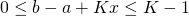Look at the right side of this inequality. We use “less or equal” operator because this is what ILP requires. We also subtract one from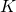because this is the scale of our numbers. Imagine now that we allow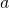and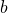to have non-integer values, e.g.,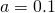and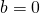. We get the following:

(1)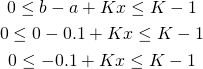Since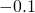is a negative number,should be true. However, in that case we get: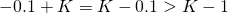This means that our formula cannot be satisfied.

# Solution 1

Once again we need to make an assumption about values of our variables. We already assumed that they cannot be greater thanand we silently assumed that the difference of two different values can be at least one. This doesn’t hold for real values so we need to verify our assumptions.
Let’s say that we want to perform exact calculations up to five digits after the decimal point. This means that the scale of our variables is equal to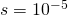. In order to compare values we need to modify formula in the following way: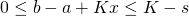Let’s now consider the situation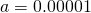and. We get:

(2)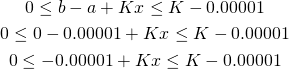Now everything works because for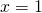we get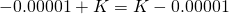. All other comparisons goes exactly the same way.

# Solution 2

We can solve the problem using another approach. Assuming that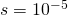we can multiply every variable by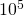and perform all calculations using only formulas for integer values. When retrieving the final results we need to divide every variable byand then we get the actual value. However, this approach is a bit more difficult because we need to remember which values were multiplied and which weren’t — we don’t want to multiply binary variables because our formulas are not adjusted for that situation.

# Warning

Both formulas are very dangerous. They assume we’ll never try comparing numbers which are not equal and are closer than epsilon. Otherwise we break the model.

Let’s consider this example. Set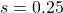meaning that our precision is one fourth. Now let’s compareand. What we get is

(3)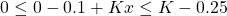Ifis zero then we get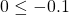which is false. However, ifis one then we get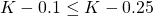.
By adding comparison (which we would like to never affect the model) we effectively break the system and solver should indicate infeasibility.

# Summary

We are now able to perform calculations using real values. As stated in summary, we should avoid this as much as possible because of rounding errors, efficiency problems, and MPS file precision. However, it is possible.